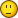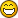# Simple pathfinding algorithm in ActionScript 3

August 6th, 2011    by sigman    13012

I was asked to quickly build an algorithm to find the shortest path for a labirinth game. First thing I did was check for some quick ideas on Wikipedia and found this article: http://en.wikipedia.org/wiki/Pathfinding. As there was only a text description I quickly wrote a simple ActionScript 3 class returning coordinates that create the shortest path.

http://sierakowski.eu/list-of-tips/88-simple-pathfinding-algorithm-in-actionscript-3.html

I won't copy the whole article from Wikipedia as you can check it out yourself but let me just copy and paste the rules of the simple method that is presented there as an example. This is the most basic algorithm, I remember learning when I was a student some more complex algorithms like Dijkstra's but this one is just enough for the basic game.

First, create a list of coordinates, which we will use as a queue. The queue will be initialized with one coordinate, the end coordinate. Each coordinate will also have a counter variable attached (the purpose of this will soon become evident). Thus, the queue starts off as ((3,8,0)).
Then, go through every element in the queue, including elements added to the end over the course of the algorithm, and to each element, do the following:
1. Create a list of the four adjacent cells, with a counter variable of the current element's counter variable + 1 (in our example, the four cells are ((2,8,1),(3,7,1),(4,8,1),(3,9,1)))
2. Check all cells in each list for the following two conditions:
- If the cell is a wall, remove it from the list
- If there is an element in the main list with the same coordinate and an equal or lower counter, remove it from the list
3. Add all remaining cells in the list to the end of the main list
4. Go to the next item in the list

And below is my implementation:

 ```1 2 3 4 5 6 7 8 9 10 11 12 13 14 15 16 17 18 19 20 21 22 23 24 25 26 27 28 29 30 31 32 33 34 35 36 37 38 39 40 41 42 43 44 45 46 47 48 49 50 51 52 53 54 55 56 57 58 59 60 61 62 63 64 65 66 67 68 69 70 71 72 73 74 75 76 77 78 79 80 81 82 83 84 85 86 87 88 89 90 91 92 93 94 95 96 97 98 99 100 101 102 103 104 105 106 107 108 109 110 111 112 113 114 115 116 117 118 119 120 121 ``` ```package { /** * ... * @author wojciech[at]sierakowski.eu * http://en.wikipedia.org/wiki/Pathfinding */ public class PathFinder { //types of map elements public static var MAP_WALL :String = "X"; public static var MAP_OPEN_SPACE :String = "_"; public static var MAP_START :String = "S"; public static var MAP_FINISH :String = "0"; public static var _map:Array, _queue:Array; public function PathFinder() { } public static function findPath(map:Array):Array { var i:int, j:int; _queue = new Array(); _map = map; //first find the coordinate of finish which is a first object var finishObject:Object; var counter:int = 0; for (i = 0; i < _map.length; i++) { for (j = 0; j < _map[i].length; j++) { if (_map[i][j] == MAP_FINISH) { finishObject = {i:i, j:j, counter:0}; break; } } } _queue.push(finishObject); //run recursive function to find the shortest path checkQueue(0, 1); return _queue; } private static function checkQueue(startIndex:int, counter:int):void { var lastQueueLength:int = _queue.length; var i:int; //check neigbours of the _queue element for (i = startIndex; i < lastQueueLength; i++) { var coordinate:Object; //check top if (_queue[i].j != 0 && _map[_queue[i].i][_queue[i].j - 1] != MAP_WALL) { coordinate = {i: _queue[i].i, j: _queue[i].j - 1, counter:counter}; //if this coordinate is the start finish algorothm as //the shortest path was just found if (_map[coordinate.i][coordinate.j] == MAP_START) return; //if a coordinate already exists in the queue //and has higher coordinate it won't be added //but if it has lower coordinate it will replace the one in the queue if (canBeAddedToQueue(coordinate)) _queue.push(coordinate); } //check right if (_queue[i].i != _map.length - 1 && _map[_queue[i].i + 1][_queue[i].j] != MAP_WALL) { coordinate = {i: _queue[i].i + 1, j: _queue[i].j, counter:counter}; if (_map[coordinate.i][coordinate.j] == MAP_START) return; if (canBeAddedToQueue(coordinate)) _queue.push(coordinate); } //check bottom if (_queue[i].j != _map[_queue[i].i].length - 1 && _map[_queue[i].i][_queue[i].j + 1] != MAP_WALL) { coordinate = {i: _queue[i].i, j: _queue[i].j + 1, counter:counter}; if (_map[coordinate.i][coordinate.j] == MAP_START) return; if (canBeAddedToQueue(coordinate)) _queue.push(coordinate); } //check left if (_queue[i].i != 0 && _map[_queue[i].i - 1][_queue[i].j] != MAP_WALL) { coordinate = {i: _queue[i].i - 1, j: _queue[i].j, counter:counter}; if (_map[coordinate.i][coordinate.j] == MAP_START) return; if (canBeAddedToQueue(coordinate)) _queue.push(coordinate); } } checkQueue(lastQueueLength, counter + 1); } public static function canBeAddedToQueue(coordinate:Object):Boolean { for (var i:int = _queue.length - 1; i >= 0 ; i--) { if (coordinate.i == _queue[i].i && coordinate.j == _queue[i].j) { if (coordinate.counter >= _queue[i].counter) { return false; } else { _queue.splice(i, 1); return true; } } } return true; } } }```

And with this code you can test it. The labirinth is exactly the same as in the Wikipedia example (the result as well):

 ```1 2 3 4 5 6 7 8 9 10 11 12 13 14 15 16 17 18 19 20 21 22 23 24 25 26 27 28 29 30 31 32 33 34 35 36 37 38 39 40 41 42 43 44 45 46 47 48 49 50 51 52 53 54 55 56 57 58 59 60 61 62 63 64 65 66 67 68 69 70 71 72 73 74 75 76 77 78 79 80 81 82 83 84 85 86 87 88 89 90 91 92 93 94 95 ``` ```package { /** * ... * @author wojciech[at]sierakowski.eu */ import flash.display.Sprite; import flash.text.TextField; public class Pathfinding extends Sprite { var map:Array; public static var MAP_ELEMENT_SIZE :int = 20; var mapSpriteBefore:Sprite, mapSpriteAfter:Sprite; public function Pathfinding() { //create a map map = new Array(); map = "X X X X X X X X X X".split(" "); map = "X _ _ _ X X _ X _ X".split(" "); map = "X _ X _ _ X _ _ _ X".split(" "); map = "X S X X _ _ _ X _ X".split(" "); map = "X _ X _ _ X _ _ _ X".split(" "); map = "X _ _ _ X X _ X _ X".split(" "); map = "X _ X _ _ X _ X _ X".split(" "); map = "X _ X X _ _ _ X _ X".split(" "); map = "X _ _ 0 _ X _ _ _ X".split(" "); map = "X X X X X X X X X X".split(" "); mapSpriteBefore = createVisualMap(map); addChild(mapSpriteBefore); var paths:Array = PathFinder.findPath(map); mapSpriteAfter = createVisualMap(map, paths); mapSpriteAfter.x = MAP_ELEMENT_SIZE * 11; addChild(mapSpriteAfter); } public function createVisualMap(mapArray:Array, paths:Array = null):Sprite { var i:int; var mapSprite:Sprite = new Sprite(); for (i = 0; i < mapArray.length; i++) { for (var j:int = 0; j < mapArray[i].length; j++) { var mapElement:Sprite = new Sprite(); switch (mapArray[i][j]) { case PathFinder.MAP_WALL: mapElement.graphics.beginFill(0x000000); mapElement.graphics.drawRect(0, 0, MAP_ELEMENT_SIZE, MAP_ELEMENT_SIZE); mapElement.graphics.endFill(); break; case PathFinder.MAP_OPEN_SPACE: mapElement.graphics.beginFill(0xFFFFFF); mapElement.graphics.drawRect(0, 0, MAP_ELEMENT_SIZE, MAP_ELEMENT_SIZE); mapElement.graphics.endFill(); break; case PathFinder.MAP_START: mapElement.graphics.beginFill(0x00FFFF); mapElement.graphics.drawRect(0, 0, MAP_ELEMENT_SIZE, MAP_ELEMENT_SIZE); mapElement.graphics.endFill(); break; case PathFinder.MAP_FINISH: mapElement.graphics.beginFill(0xFF0000, .5); mapElement.graphics.drawRect(0, 0, MAP_ELEMENT_SIZE, MAP_ELEMENT_SIZE); mapElement.graphics.endFill(); break; } mapElement.x = j * MAP_ELEMENT_SIZE; mapElement.y = i * MAP_ELEMENT_SIZE; mapSprite.addChild(mapElement); } } if (paths != null) { for (i = 0; i < paths.length; i++) { var label_txt:TextField = new TextField(); label_txt.text = paths[i].counter; label_txt.x = paths[i].j * MAP_ELEMENT_SIZE; label_txt.y = paths[i].i * MAP_ELEMENT_SIZE; mapSprite.addChild(label_txt); } } return mapSprite; } } }```

And now quoting the Wikipedia's article: "Now, start at Start and go to the nearby cell with the lowest number (unchecked cells cannot be moved to).".

No doubt there might be some more optimal way of doing this, but this one does the job alright ;) If you have any suggestion or came up with other (and better) algorithms in ActionScript and would like to share it with the community, add a comment with a link to your blog article.

#### Comments imported from the original article @sierakowski.eu

+- 0 #5 Hari 2014-05-10 06:52i have no errors but nothing is displayed on my screen. can you please help me how will i can compile this .as files. Am very new to AS3. Thanks in Advance!!

+- +1 #4 ehaugw 2013-07-11 22:07
Isn't this what's called the A* algorithm?

+- 0 #3 David J. 2013-05-17 18:10
I'm glad I finally found a pathfinding algorithm that doesn't take hours to understand. Thanks a ton for sharing this!

+- 0 #2 Alex 2012-07-21 22:42
I know this article is almost a year old, but I love it! It's thought through correctly and is overall a fascinating approach+- +1 #1 Aubrius 2011-12-14 22:55
I have used other people's algorithms and functions for path-finding before, but I never understood the purpose of the queue until I read this post. Thanks for sharing it!